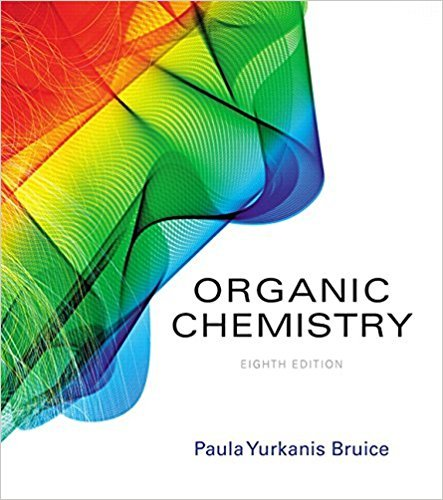×
×

# Solutions for Chapter 3.5: The Nomenclature of Ethers## Full solutions for Organic Chemistry | 8th Edition

ISBN: 9780134042282Solutions for Chapter 3.5: The Nomenclature of Ethers

Solutions for Chapter 3.5
4 5 0 318 Reviews
19
4
##### ISBN: 9780134042282

This expansive textbook survival guide covers the following chapters and their solutions. Organic Chemistry was written by and is associated to the ISBN: 9780134042282. Chapter 3.5: The Nomenclature of Ethers includes 5 full step-by-step solutions. Since 5 problems in chapter 3.5: The Nomenclature of Ethers have been answered, more than 33384 students have viewed full step-by-step solutions from this chapter. This textbook survival guide was created for the textbook: Organic Chemistry, edition: 8.

Key Chemistry Terms and definitions covered in this textbook
• activation energy (Ea).

The minimum amount of energy required to initiate a chemical reaction. (13.4)

• Allylic carbon

A carbon adjacent to a carbon-carbon double bond.

• battery

A self-contained electrochemical power source that contains one or more voltaic cells. (Section 20.7)

• beta particles.

See beta rays.

• Bonding molecular orbital

A molecular orbital in which electrons have a lower energy than they would in isolated atomic orbitals

• Charles’s law

A law stating that at constant pressure, the volume of a given quantity of gas is proportional to absolute temperature. (Section 10.3)

• chemical equation.

An equation that uses chemical symbols to show what happens during a chemical reaction. (3.7)

• dextrorotatory, or merely dextro or d

A term used to label a chiral molecule that rotates the plane of polarization of plane-polarized light to the right (clockwise). (Section 23.4)

• diazonium salt

An ionic compound that is formed upon treatment of a primary amine with NaNO2 and HCl.

• Dielectric constant

A measure of a solvent’s ability to insulate opposite charges from one another

• Disaccharide

A carbohydrate containing two monosaccharide units joined by a glycosidic bond.

• dynamic equilibrium

A state of balance in which opposing processes occur at the same rate. (Section 11.5)

• Fatty acid

A long, unbranched-chain carboxylic acid, most commonly of 12 to 20 carbons, derived from the hydrolysis of animal fats, vegetable oils, or the phospholipids of biological membranes.

• heterogeneous equilibrium

The equilibrium established between substances in two or more different phases, for example, between a gas and a solid or between a solid and a liquid. (Section 15.4)

• lattice vectors

The vectors a, b, and c that define a crystal lattice. The position of any lattice point in a crystal can be represented by summing integer multiples of the lattice vectors. (Section 12.2)

• neutron

An electrically neutral particle found in the nucleus of an atom; it has approximately the same mass as a proton. (Section 2.3)

• Plane of symmetry

An imaginary plane passing through an object dividing it so that one half is the mirror image of the other half

• rate-determining step

The slowest elementary step in a reaction mechanism. (Section 14.6)

• Resonance in NMR spectroscopy

The absorption of electromagnetic radiation by a precessing nucleus and the resulting “fl ip” of its nuclear spin from the lower energy state to the higher energy state.

• resonance structures

A series of structures that are melded together (conceptually) to circumvent the inadequacies of bond-line drawings.

×